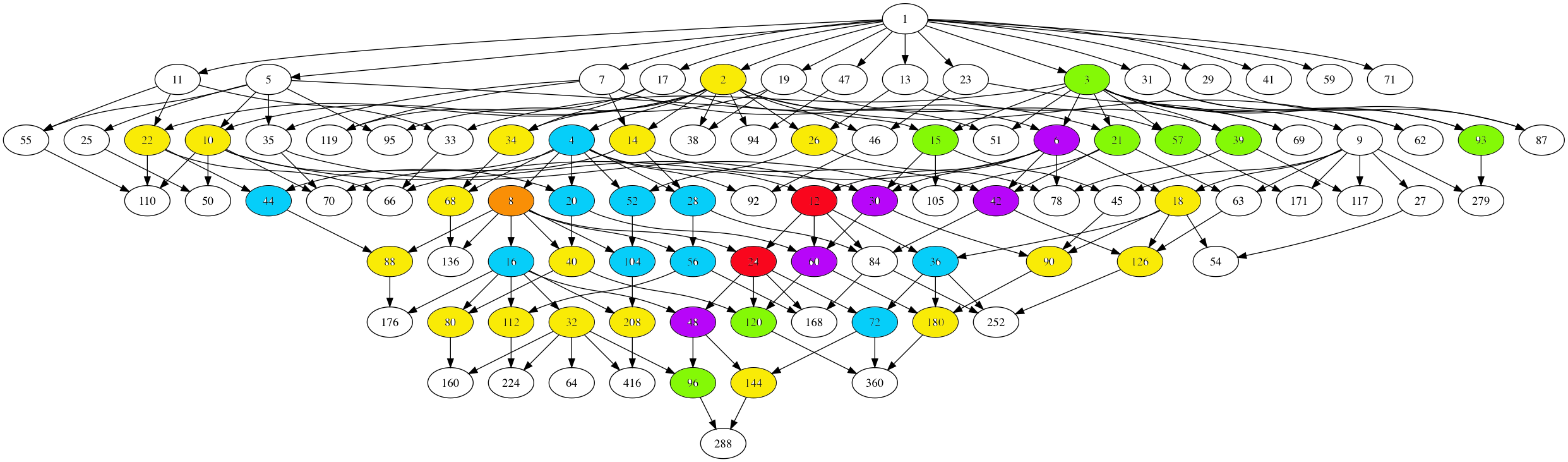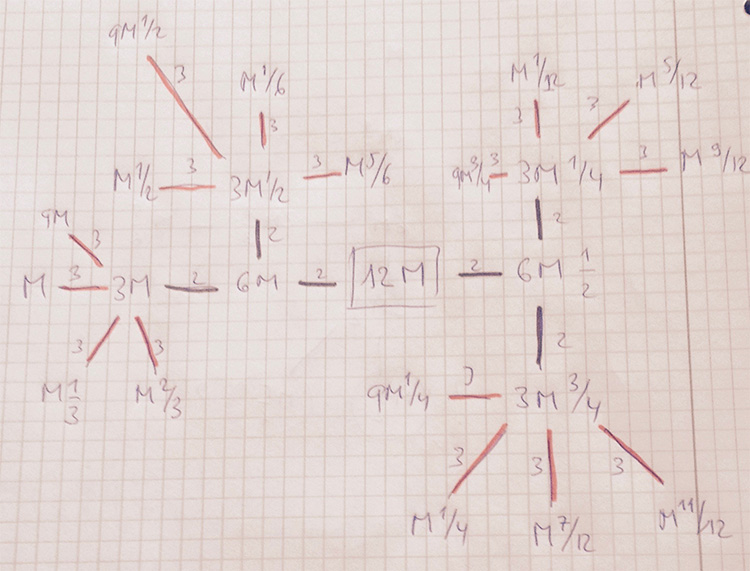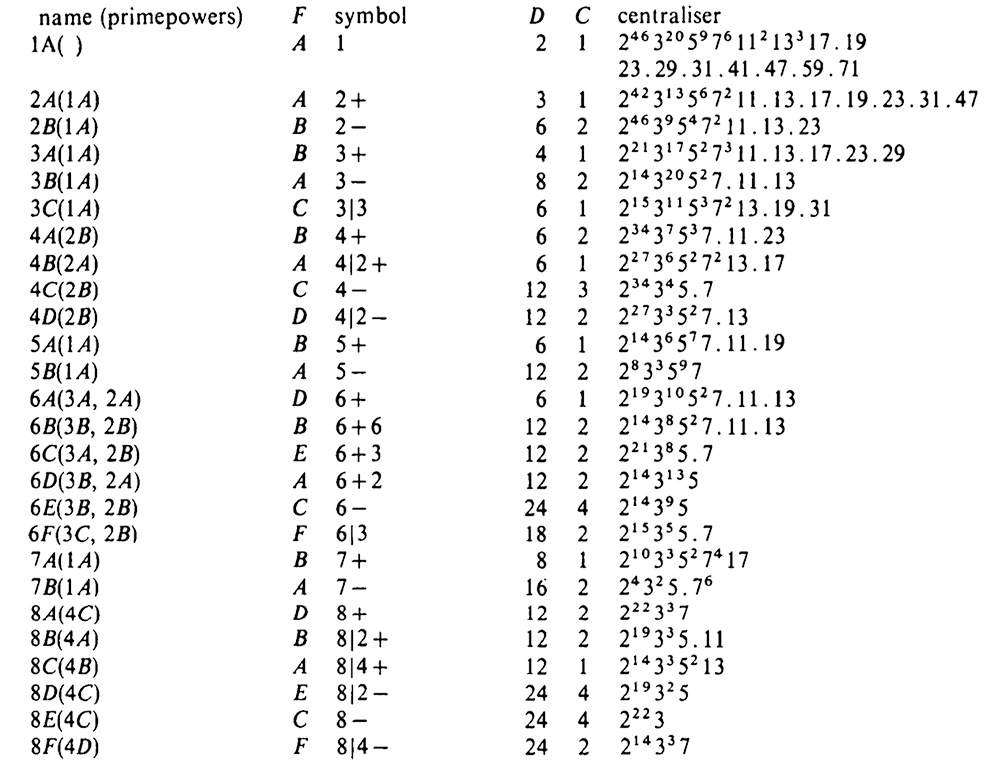# Tag: monster

The monstrous moonshine picture is the subgraph of Conway’s big picture consisting of all lattices needed to describe the 171 moonshine groups.

It consists of:

– exactly 218 vertices (that is, lattices), out of which

– 97 are number-lattices (that is of the form $M$ with $M$ a positive integer), and

– 121 are proper number-like lattices (that is of the form $M \frac{g}{h}$ with $M$ a positive integer, $h$ a divisor of $24$ and $1 \leq g \leq h$ with $(g,h)=1$).

The $97$ number lattices are closed under taking divisors, and the corresponding Hasse diagram has the following shapeHere, number-lattices have the same colour if they have the same local structure in the moonshine picture (that is, have a similar neighbourhood of proper number-like lattices).

There are 7 different types of local behaviour:

The white numbered lattices have no proper number-like neighbours in the picture.

The yellow number lattices (2,10,14,18,22,26,32,34,40,68,80,88,90,112,126,144,180,208 = 2M) have local structure

$\xymatrix{M \ar@{-}[r] & \color{yellow}{2M} \ar@{-}[r] & M \frac{1}{2}}$

which involves all $2$-nd (square) roots of unity centered at the lattice.

The green number lattices (3,15,21,39,57,93,96,120 = 3M) have local structure

$\xymatrix{& M \ar@[red]@{-}[d] & \\ M \frac{1}{3} \ar@[red]@{-}[r] & \color{green}{3M} \ar@[red]@{-}[r] & M \frac{2}{3}}$

which involve all $3$-rd roots of unity centered at the lattice.

The blue number lattices (4,16,20,28,36,44,52,56,72,104 = 4M) have as local structure

$\xymatrix{M \frac{1}{2} \ar@{-}[d] & & M \frac{1}{4} \ar@{-}[d] \\ 2M \ar@{-}[r] & \color{blue}{4M} \ar@{-}[r] & 2M \frac{1}{2} \ar@{-}[d] \\ M \ar@{-}[u] & & M \frac{3}{4}}$

and involve the $2$-nd and $4$-th root of unity centered at the lattice.

The purple number lattices (6,30,42,48,60 = 6M) have local structure

$\xymatrix{& M \frac{1}{3} \ar@[red]@{-}[d] & 2M \frac{1}{3} & M \frac{1}{6} \ar@[red]@{-}[d] & \\ M \ar@[red]@{-}[r] & 3M \ar@{-}[r] \ar@[red]@{-}[d] & \color{purple}{6M} \ar@{-}[r] \ar@[red]@{-}[u] \ar@[red]@{-}[d] & 3M \frac{1}{2} \ar@[red]@{-}[r] \ar@[red]@{-}[d] & M \frac{5}{6} \\ & M \frac{2}{3} & 2M \frac{2}{3} & M \frac{1}{2} & }$

and involve all $2$-nd, $3$-rd and $6$-th roots of unity centered at the lattice.

The unique brown number lattice 8 has local structure

$\xymatrix{& & 1 \frac{1}{4} \ar@{-}[d] & & 1 \frac{1}{8} \ar@{-}[d] & \\ & 1 \frac{1}{2} \ar@{-}[d] & 2 \frac{1}{2} \ar@{-}[r] \ar@{-}[d] & 1 \frac{3}{4} & 2 \frac{1}{4} \ar@{-}[r] & 1 \frac{5}{8} \\ 1 \ar@{-}[r] & 2 \ar@{-}[r] & 4 \ar@{-}[r] & \color{brown}{8} \ar@{-}[r] & 4 \frac{1}{2} \ar@{-}[d] \ar@{-}[u] & \\ & & & 1 \frac{7}{8} \ar@{-}[r] & 2 \frac{3}{4} \ar@{-}[r] & 1 \frac{3}{8}}$

which involves all $2$-nd, $4$-th and $8$-th roots of unity centered at $8$.

Finally, the local structure for the central red lattices $12,24 = 12M$ is

$\xymatrix{ M \frac{1}{12} \ar@[red]@{-}[dr] & M \frac{5}{12} \ar@[red]@{-}[d] & M \frac{3}{4} \ar@[red]@{-}[dl] & & M \frac{1}{6} \ar@[red]@{-}[dr] & M \frac{1}{2} \ar@[red]@{-}[d] & M \frac{5}{6} \ar@[red]@{-}[dl] \\ & 3M \frac{1}{4} \ar@{-}[dr] & 2M \frac{1}{6} \ar@[red]@{-}[d] & 4M \frac{1}{3} \ar@[red]@{-}[d] & 2M \frac{1}{3} \ar@[red]@{-}[d] & 3M \frac{1}{2} \ar@{-}[dl] & \\ & 2M \frac{1}{2} \ar@[red]@{-}[r] & 6M \frac{1}{2} \ar@{-}[dl] \ar@[red]@{-}[d] \ar@{-}[r] & \color{red}{12M} \ar@[red]@{-}[d] \ar@{-}[r] & 6M \ar@[red]@{-}[d] \ar@{-}[dr] \ar@[red]@{-}[r] & 2M & \\ & 3M \frac{3}{4} \ar@[red]@{-}[dl] \ar@[red]@{-}[d] \ar@[red]@{-}[dr] & 2M \frac{5}{6} & 4M \frac{2}{3} & 2M \frac{2}{3} & 3M \ar@[red]@{-}[dl] \ar@[red]@{-}[d] \ar@[red]@{-}[dr] & \\ M \frac{1}{4} & M \frac{7}{12} & M \frac{11}{12} & & M \frac{1}{3} & M \frac{2}{3} & M}$

It involves all $2$-nd, $3$-rd, $4$-th, $6$-th and $12$-th roots of unity with center $12M$.

No doubt this will be relevant in connecting moonshine with non-commutative geometry and issues of replicability as in Plazas’ paper Noncommutative Geometry of Groups like $\Gamma_0(N)$.

Another of my pet follow-up projects is to determine whether or not the monster group $\mathbb{M}$ dictates the shape of the moonshine picture.

That is, can one recover the 97 number lattices and their partition in 7 families starting from the set of element orders of $\mathbb{M}$, applying some set of simple rules?

One of these rules will follow from the two equivalent notations for lattices, and the two different sets of roots of unities centered at a given lattice. This will imply that if a number lattice belongs to a given family, certain divisors and multiples of it must belong to related families.

If this works out, it may be a first step towards a possibly new understanding of moonshine.

The largest snake in the moonshine picture determines the moonshine group $(24|12)$ and is associated to conjugacy class $24J$ of the monster.

It contains $70$ lattices, about one third of the total number of lattices in the moonshine picture.

The anaconda’s backbone is the $(288|1)$ thread below (edges in the $2$-tree are black, those in the $3$-tree red and coloured numbers are symmetric with respect to the $(24|12)$-spine and have the same local snake-structure.

$\xymatrix{9 \ar@{-}[r] \ar@[red]@{-}[d] & \color{green}{18} \ar@{-}[r] \ar@[red]@{-}[d] & \color{yellow}{36} \ar@{-}[r] \ar@[red]@{-}[d] & \color{yellow}{72} \ar@{-}[r] \ar@[red]@{-}[d] & \color{green}{144} \ar@{-}[r] \ar@[red]@{-}[d] & 288 \ar@[red]@{-}[d] \\ 3 \ar@{-}[r] \ar@[red]@{-}[d] & \color{blue}{6} \ar@{-}[r] \ar@[red]@{-}[d] & \color{red}{12} \ar@{-}[r] \ar@[red]@{-}[d] & \color{red}{24} \ar@{-}[r] \ar@[red]@{-}[d] & \color{blue}{48} \ar@{-}[r] \ar@[red]@{-}[d] & 96 \ar@[red]@{-}[d] \\ 1 \ar@{-}[r] & \color{green}{2} \ar@{-}[r] & \color{yellow}{4} \ar@{-}[r] & \color{yellow}{8} \ar@{-}[r] & \color{green}{16} \ar@{-}[r] & 32 }$

These are the only number-lattices in the anaconda. The remaining lattices are number-like, that is of the form $M \frac{g}{h}$ with $M$ an integer and $1 \leq g < h$ with $(g,h)=1$.
There are

– $12$ with $h=2$ and $M$ a divisor of $72$.

– $12$ with $h=3$ and $M$ a divisor of $32$.

– $12$ with $h=4$ and $M$ a divisor of $18$.

– $8$ with $h=6$ and $M$ a divisor of $8$.

– $8$ with $h=12$ and $M=1,2$.

The non-number lattices in the snake are locally in the coloured numbers:

In $2,16,18,144=2M$

$\xymatrix{& \color{green}{2M} \ar@{-}[r] & M \frac{1}{2}}$

In $4,8,36,72=4M$

$\xymatrix{M \frac{1}{2} \ar@{-}[d] & & M \frac{1}{4} \ar@{-}[d] \\ 2M \ar@{-}[r] & \color{yellow}{4M} \ar@{-}[r] & 2M \frac{1}{2} \ar@{-}[d] \\ & & M \frac{3}{4}}$

In $6,48=6M$

$\xymatrix{M \frac{1}{3} \ar@[red]@{-}[d] & 2M \frac{1}{3} & M \frac{1}{6} \ar@[red]@{-}[d] & \\ 3M \ar@{-}[r] \ar@[red]@{-}[d] & \color{blue}{6M} \ar@{-}[r] \ar@[red]@{-}[u] \ar@[red]@{-}[d] & 3M \frac{1}{2} \ar@[red]@{-}[r] \ar@[red]@{-}[d] & M \frac{5}{6} \\ M \frac{2}{3} & 2M \frac{2}{3} & M \frac{1}{2} & }$

In $12,24=12M$ the local structure looks likeHere, we used the commutation relations to reach all lattices at distance $log(6)$ and $log(12)$ by first walking the $2$-adic tree and postpone the last step for the $3$-tree.

Perhaps this is also a good strategy to get a grip on the full moonshine picture:

First determine subsets of the moonshine thread with the same local structure, and then determine for each class this local structure.

Monstrous moonshine associates to every element of order $n$ of the monster group $\mathbb{M}$ an arithmetic group of the form
$(n|h)+e,f,\dots$
where $h$ is a divisor of $24$ and of $n$ and where $e,f,\dots$ are divisors of $\frac{n}{h}$ coprime with its quotient.

In snakes, spines, and all that we’ve constructed the arithmetic group
$\Gamma_0(n|h)+e,f,\dots$
which normalizes $\Gamma_0(N)$ for $N=h.n$. If $h=1$ then this group is the moonshine group $(n|h)+e,f,\dots$, but for $h > 1$ the moonshine group is a specific subgroup of index $h$ in $\Gamma_0(n|h)+e,f,\dots$.

I’m sure one can describe this subgroup explicitly in each case by analysing the action of the finite group $(\Gamma_0(n|h)+e,f,\dots)/\Gamma_0(N)$ on the $(N|1)$-snake. Some examples were worked out by John Duncan in his paper Arithmetic groups and the affine E8 Dynkin diagram.

But at the moment I don’t understand the general construction given by Conway, McKay and Sebbar in On the discrete groups of moonshine. I’m stuck at the last sentence of (2) in section 3. Nothing a copy of Charles Ferenbaugh Ph. D. thesis cannot fix.

The correspondence between the conjugacy classes of the Monster and these arithmetic groups takes up 3 pages in Conway & Norton’s Monstrous Moonshine. Here’s the beginning of it.Conway introduced his Big Picture to make it easier to understand and name the groups appearing in Monstrous Moonshine.

For $M \in \mathbb{Q}_+$ and $0 \leq \frac{g}{h} < 1$, $M,\frac{g}{h}$ denotes (the projective equivalence class of) the lattice $\mathbb{Z} (M \vec{e}_1 + \frac{g}{h} \vec{e}_2) \oplus \mathbb{Z} \vec{e}_2$ which we also like to represent by the $2 \times 2$ matrix $\alpha_{M,\frac{g}{h}} = \begin{bmatrix} M & \frac{g}{h} \\ 0 & 1 \end{bmatrix}$ A subgroup $G$ of $GL_2(\mathbb{Q})$ is said to fix $M,\frac{g}{h}$ if
$\alpha_{M,\frac{g}{h}}.G.\alpha_{M,\frac{g}{h}}^{-1} \subset SL_2(\mathbb{Z})$
The full group of all elements fixing $M,\frac{g}{h}$ is the conjugate
$\alpha_{M,\frac{g}{h}}^{-1}.SL_2(\mathbb{Z}).\alpha_{M,\frac{g}{h}}$
For a number lattice $N=N,0$ the elements of this group are all of the form
$\begin{bmatrix} a & \frac{b}{N} \\ cN & d \end{bmatrix} \qquad \text{with} \qquad \begin{bmatrix} a & b \\ c & d \end{bmatrix} \in SL_2(\mathbb{Z})$
and the intersection with $SL_2(\mathbb{Z})$ (which is the group of all elements fixing the lattice $1=1,0$) is the congruence subgroup
$\Gamma_0(N) = \{ \begin{bmatrix} a & b \\ cN & d \end{bmatrix}~|~ad-Nbc = 1 \}$
Conway argues that this is the real way to think of $\Gamma_0(N)$, as the joint stabilizer of the two lattices $N$ and $1$!

The defining definition of 24 tells us that $\Gamma_0(N)$ fixes more lattices. In fact, it fixes exactly the latices $M \frac{g}{h}$ such that
$1~|~M~|~\frac{N}{h^2} \quad \text{with} \quad h^2~|~N \quad \text{and} \quad h~|~24$
Conway calls the sub-graph of the Big Picture on these lattices the snake of $(N|1)$.

Here’s the $(60|1)$-snake (note that $60=2^2.3.5$ so $h=1$ or $h=2$ and edges corresponding to the prime $2$ are coloured red, those for $3$ green and for $5$ blue).

$\xymatrix{& & & 15 \frac{1}{2} \ar@[red]@{-}[dd] & & \\ & & 5 \frac{1}{2} \ar@[red]@{-}[dd] & & & \\ & 15 \ar@[red]@{-}[rr] \ar@[blue]@{-}[dd] & & 30 \ar@[red]@{-}[rr] \ar@[blue]@{-}[dd] & & 60 \ar@[blue]@{-}[dd] \\ 5 \ar@[green]@{-}[ru] \ar@[blue]@{-}[dd] \ar@[red]@{-}[rr] & & 10 \ar@[green]@{-}[ru] \ar@[red]@{-}[rr] \ar@[blue]@{-}[dd] & & 20 \ar@[green]@{-}[ru] \ar@[blue]@{-}[dd] & \\ & 3 \ar@[red]@{-}[rr] & & 6 \ar@[red]@{-}[rr] \ar@[red]@{-}[dd] & & 12 \\ 1 \ar@[green]@{-}[ru] \ar@[red]@{-}[rr] & & 2 \ar@[green]@{-}[ru] \ar@[red]@{-}[rr] \ar@[red]@{-}[dd] & & 4 \ar@[green]@{-}[ru] & \\ & & & 3\frac{1}{2} & & \\ & & 1 \frac{1}{2} & & &}$

The sub-graph of lattices fixed by $\Gamma_0(N)$ for $h=1$, that is all number-lattices $M=M,0$ for $M$ a divisor of $N$ is called the thread of $(N|1)$. Here’s the $(60|1)$-thread

$\xymatrix{ & 15 \ar@[red]@{-}[rr] \ar@[blue]@{-}[dd] & & 30 \ar@[red]@{-}[rr] \ar@[blue]@{-}[dd] & & 60 \ar@[blue]@{-}[dd] \\ 5 \ar@[green]@{-}[ru] \ar@[blue]@{-}[dd] \ar@[red]@{-}[rr] & & 10 \ar@[green]@{-}[ru] \ar@[red]@{-}[rr] \ar@[blue]@{-}[dd] & & 20 \ar@[green]@{-}[ru] \ar@[blue]@{-}[dd] & \\ & 3 \ar@[red]@{-}[rr] & & 6 \ar@[red]@{-}[rr] & & 12 \\ 1 \ar@[green]@{-}[ru] \ar@[red]@{-}[rr] & & 2 \ar@[green]@{-}[ru] \ar@[red]@{-}[rr] & & 4 \ar@[green]@{-}[ru] & }$

If $N$ factors as $N = p_1^{e_1} p_2^{e_2} \dots p_k^{e_k}$ then the $(N|1)$-thread is the product of the $(p_i^{e_i}|1)$-threads and has a symmetry group of order $2^k$.

It is generated by $k$ involutions, each one the reflexion in one $(p_i^{e_i}|1)$-thread and the identity on the other $(p_j^{e_j}|1)$-threads.
In the $(60|1)$-thread these are the reflexions in the three mirrors of the figure.

So, there is one involution for every divisor $e$ of $N$ such that $(e,\frac{N}{e})=1$. For such an $e$ there are matrices, with $a,b,c,d \in \mathbb{Z}$, of the form
$W_e = \begin{bmatrix} ae & b \\ cN & de \end{bmatrix} \quad \text{with} \quad ade^2-bcN=e$
Think of Bezout and use that $(e,\frac{N}{e})=1$.

Such $W_e$ normalizes $\Gamma_0(N)$, that is, for any $A \in \Gamma_0(N)$ we have that $W_e.A.W_e^{-1} \in \Gamma_0(N)$. Also, the determinant of $W_e^e$ is equal to $e^2$ so we can write $W_e^2 = e A$ for some $A \in \Gamma_0(N)$.

That is, the transformation $W_e$ (left-multiplication) sends any lattice in the thread or snake of $(N|1)$ to another such lattice (up to projective equivalence) and if we apply $W_e^2$ if fixes each such lattice (again, up to projective equivalence), so it is the desired reflexion corresponding with $e$.

Consider the subgroup of $GL_2(\mathbb{Q})$ generated by $\Gamma_0(N)$ and some of these matrices $W_e,W_f,\dots$ and denote by $\Gamma_0(N)+e,f,\dots$ the quotient modulo positive scalar matrices, then
$\Gamma_0(N) \qquad \text{is a normal subgroup of} \qquad \Gamma_0(N)+e,f,\dots$
with quotient isomorphic to some $(\mathbb{Z}/2\mathbb{Z})^l$ isomorphic to the subgroup generated by the involutions corresponding to $e,f,\dots$.

More generally, consider the $(n|h)$-thread for number lattices $n=n,0$ and $h=h,0$ such that $h | n$ as the sub-graph on all number lattices $l=l,0$ such that $h | l | n$. If we denote with $\Gamma_0(n|h)$ the point-wise stabilizer of $n$ and $h$, then we have that
$\Gamma(n|h) = \begin{bmatrix} h & 0 \\ 0 & 1 \end{bmatrix}^{-1}.\Gamma_0(\frac{n}{h}).\begin{bmatrix} h & 0 \\ 0 & 1 \end{bmatrix}$
and we can then denote with
$\Gamma_0(n|h)+e,f,\dots$
the conjugate of the corresponding group $\Gamma_0(\frac{n}{h})+e,f,\dots$.

If $h$ is the largest divisor of $24$ such that $h^2$ divides $N$, then Conway calls the spine of the $(N|1)$-snake the subgraph on all lattices of the snake whose distance from its periphery is exactly $log(h)$.

For $N=60$, $h=2$ and so the spine of the $(60|1)$-snake is the central piece connected with double black edges

$\xymatrix{& & & 15 \frac{1}{2} \ar@[red]@{-}[dd] & & \\ & & 5 \frac{1}{2} \ar@[red]@{-}[dd] & & & \\ & 15 \ar@[red]@{-}[rr] \ar@[blue]@{-}[dd] & & 30 \ar@[red]@{-}[rr] \ar@[black]@{=}[dd] & & 60 \ar@[blue]@{-}[dd] \\ 5 \ar@[green]@{-}[ru] \ar@[blue]@{-}[dd] \ar@[red]@{-}[rr] & & 10 \ar@[black]@{=}[ru] \ar@[red]@{-}[rr] \ar@[black]@{=}[dd] & & 20 \ar@[green]@{-}[ru] \ar@[blue]@{-}[dd] & \\ & 3 \ar@[red]@{-}[rr] & & 6 \ar@[red]@{-}[rr] \ar@[red]@{-}[dd] & & 12 \\ 1 \ar@[green]@{-}[ru] \ar@[red]@{-}[rr] & & 2 \ar@[black]@{=}[ru] \ar@[red]@{-}[rr] \ar@[red]@{-}[dd] & & 4 \ar@[green]@{-}[ru] & \\ & & & 3\frac{1}{2} & & \\ & & 1 \frac{1}{2} & & &}$

which is the $(30|2)$-thread.

The upshot of all this is to have a visual proof of the Atkin-Lehner theorem which says that the full normalizer of $\Gamma_0(N)$ is the group $\Gamma_0(\frac{N}{h}|h)+$ (that is, adding all involutions) where $h$ is the largest divisor of $24$ for which $h^2|N$.

Any element of this normalizer must take every lattice in the $(N|1)$-snake fixed by $\Gamma_0(N)$ to another such lattice. Thus it follows that it must take the snake to itself.
Conversely, an element that takes the snake to itself must conjugate into itself the group of all matrices that fix every point of the snake, that is to say, must normalize $\Gamma_0(N)$.

But the elements that take the snake to itself are precisely those that take the spine to itself, and since this spine is just the $(\frac{N}{h}|h)$-thread, this group is just $\Gamma_0(\frac{N}{h}|h)+$.

Reference: J.H. Conway, “Understanding groups like $\Gamma_0(N)$”, in “Groups, Difference Sets, and the Monster”, Walter de Gruyter-Berlin-New York, 1996

From Wikipedia on 24:

“$24$ is the only number whose divisors, namely $1, 2, 3, 4, 6, 8, 12, 24$, are exactly those numbers $n$ for which every invertible element of the commutative ring $\mathbb{Z}/n\mathbb{Z}$ is a square root of $1$. It follows that the multiplicative group $(\mathbb{Z}/24\mathbb{Z})^* = \{ \pm 1, \pm 5, \pm 7, \pm 11 \}$ is isomorphic to the additive group $(\mathbb{Z}/2\mathbb{Z})^3$. This fact plays a role in monstrous moonshine.”

Where did that come from?

In the original “Monstrous Moonshine” paper by John Conway and Simon Norton, section 3 starts with:

“It is a curious fact that the divisors $h$ of $24$ are precisely those numbers $h$ for which $x.y \equiv 1~(mod~h)$ implies $x \equiv y~(mod~h)$.”

and a bit further they even call this fact:

“our ‘defining property of $24$'”.The proof is pretty straightforward.

We want all $h$ such that every unit in $\mathbb{Z}/h \mathbb{Z}$ has order two.

By the Chinese remainder theorem we only have to check this for prime powers dividing $h$.

$5$ is a unit of order $4$ in $\mathbb{Z}/16 \mathbb{Z}$.

$2$ is a unit of order $6$ in $\mathbb{Z}/ 9 \mathbb{Z}$.

A generator of the cyclic group $(\mathbb{Z}/p\mathbb{Z})^*$ is a unit of order $p-1 > 2$ in $\mathbb{Z}/p \mathbb{Z}$, for any prime number $p \geq 5$.

This only leaves those $h$ dividing $2^3.3=24$.

But, what does it have to do with monstrous moonshine?

Moonshine assigns to elements of the Monster group $\mathbb{M}$ a specific subgroup of $SL_2(\mathbb{Q})$ containing a cofinite congruence subgroup

$\Gamma_0(N) = \{ \begin{bmatrix} a & b \\ cN & d \end{bmatrix}~|~a,b,c,d \in \mathbb{Z}, ad-Nbc = 1 \}$

for some natural number $N = h.n$ where $n$ is the order of the monster-element, $h^2$ divides $N$ and … $h$ is a divisor of $24$.

To begin to understand how the defining property of $24$ is relevant in this, take any strictly positive rational number $M$ and any pair of coprime natural numbers $g < h$ and associate to $M \frac{g}{h}$ the matrix $\alpha_{M\frac{g}{h}} = \begin{bmatrix} M & \frac{g}{h} \\ 0 & 1 \end{bmatrix}$ We say that $\Gamma_0(N)$ fixes $M \frac{g}{h}$ if we have that
$\alpha_{M\frac{g}{h}} \Gamma_0(N) \alpha_{M\frac{g}{h}}^{-1} \subset SL_2(\mathbb{Z})$

For those in the know, $M \frac{g}{h}$ stands for the $2$-dimensional integral lattice
$\mathbb{Z} (M \vec{e}_1 + \frac{g}{h} \vec{e}_2) \oplus \mathbb{Z} \vec{e}_2$
and the condition tells that $\Gamma_0(N)$ preserves this lattice under base-change (right-multiplication).

In “Understanding groups like $\Gamma_0(N)$” Conway describes the groups appearing in monstrous moonshine as preserving specific finite sets of these lattices.

For this, it is crucial to determine all $M\frac{g}{h}$ fixed by $\Gamma_0(N)$.

$\alpha_{M\frac{g}{h}}.\begin{bmatrix} 1 & 1 \\ 0 & 1 \end{bmatrix}.\alpha_{M\frac{g}{h}}^{-1} = \begin{bmatrix} 1 & M \\ 0 & 1 \end{bmatrix}$

so we must have that $M$ is a natural number, or that $M\frac{g}{h}$ is a number-like lattice, in Conway-speak.

$\alpha_{M\frac{g}{h}}.\begin{bmatrix} 1 & 0 \\ N & 1 \end{bmatrix}.\alpha_{M\frac{g}{h}}^{-1} = \begin{bmatrix} 1 + \frac{Ng}{Mh} & – \frac{Ng^2}{Mh^2} \\ \frac{N}{M} & 1 – \frac{Ng}{Mh} \end{bmatrix}$

so $M$ divides $N$, $Mh$ divides $Ng$ and $Mh^2$ divides $Ng^2$. As $g$ and $h$ are coprime it follows that $Mh^2$ must divide $N$.

Now, for an arbitrary element of $\Gamma_0(N)$ we have

$\alpha_{M\frac{g}{h}}.\begin{bmatrix} a & b \\ cN & d \end{bmatrix}.\alpha_{M\frac{g}{h}}^{-1} = \begin{bmatrix} a + c \frac{Ng}{Mh} & Mb – c \frac{Ng^2}{Mh^2} – (a-d) \frac{g}{h} \\ c \frac{N}{M} & d – c \frac{Ng}{Mh} \end{bmatrix}$
and using our divisibility requirements it follows that this matrix belongs to $SL_2(\mathbb{Z})$ if $a-d$ is divisible by $h$, that is if $a \equiv d~(mod~h)$.

We know that $ad-Nbc=1$ and that $h$ divides $N$, so $a.d \equiv 1~(mod~h)$, which implies $a \equiv d~(mod~h)$ if $h$ satisfies the defining property of $24$, that is, if $h$ divides $24$.

Concluding, $\Gamma_0(N)$ preserves exactly those lattices $M\frac{g}{h}$ for which
$1~|~M~|~\frac{N}{h^2}~\quad~\text{and}~\quad~h~|~24$

A first step towards figuring out the Moonshine Picture.